# Forces and Straight-Line Motion

Forces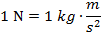Force is a measure of the interactions between objects. It has both a magnitude and a direction, and so it is a vector quantity. The SI unit of force is the Newton (N), which is defined as,

The "superposition of forces" relates to how forces add. If there are multiple forces acting on the same object, there is a total force that is equal to the vector sum of the forces. If forces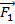,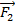, etc. act on an object, there is a total force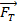equal to,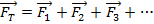The superposition of forces also means that forces can be broken into components. Any force can be treated as the vector sum of a number of other forces. Many physics problems are made simpler by separating a force into components that are perpendicular to each other. One way this can help is to find the components of the vector in the x, y, and z axes,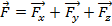If we stick to working with two dimensions, and the angle between the force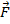and the x axis is a known value θ ("theta"), then the component forces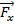and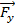are,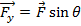The magnitude of the total forceis,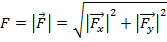The angle θ is,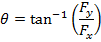Weight

In everyday speech, the terms "weight" and "mass" are often used to mean the same thing. People use these words to explain how much matter there is in something. In physics, only "mass" refers to the quantity of material in an object. Whether this mass is measured in pounds, kilograms, or other units, it is a property of an object that does not change. "Weight" is different, and relates to the way that gravity acts on an object. In physics, "weight" is a force.

The Earth's gravitational field causes objects to accelerate toward it. The acceleration due to gravity is g. This value changes at different heights above the Earth. Newton's second law states that the total force acting on an object is, where m in this equation is the mass. This means that, for an object in freefall, the force acting on it has a magnitude of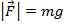. This force is the weight of the object,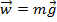The value of g at sea level is equal to 9.80 m/s2, but the value changes at different heights. Therefore, the weight that acts on the object depends on the local acceleration due to gravity, while the mass m is the same everywhere.

Normal Force

Newton's third law of motion is often stated "for every action there is an equal and opposite reaction". This means that forces come in action-reaction pairs. An object on Earth constantly experiences the force due to gravity (weight) directed downward. If this object is sitting on the ground (or a table, or other surface), even though it is pulled downward by its weight, it does not move. Why is this? If the force of gravity causing the object to push against the ground is an action force, then there must be a reaction force of the ground pushing back. This reaction is called the "normal force", and it is usually represented with the letter n, or with the Greek letter η ("eta").

The normal force is a reaction force that is always perpendicular to the surface of contact. On a flat surface, the normal force is equal in magnitude and opposite in direction to the weight of the object pushing down. However, if the surface is on an angle, the normal force will be perpendicular to the surface, and will have a smaller magnitude than the downward weight. In this case, the reaction to the downward weight is split between two components. The first is the normal force, and the second is a force that is parallel to the surface, and directed upward along it. This is the force of friction, which will be described in the next section. A total reaction force that is equal in magnitude to the downward weight can be made by summing the two reaction force components using vector addition.

If the angle between the sloped surface and the horizontal is θ, then the magnitude of the normal force (perpendicular to the surface) will be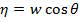Since the weight is equal to the mass of the object multiplied by the acceleration due to gravity,,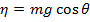The remaining component of the weight has a magnitude of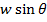. If the object is in equilibrium, and therefore all of the forces add to zero, then this is also the magnitude of the force of friction.

Friction

When an object sits or slides on an angled surface, or when a force pushes an object over a surface, a reaction force called friction resists the motion. Friction is caused by interactions between the tiny cracks, bumps, and imperfections in the surfaces that are in contact. Even on the same surface, two objects with the same mass but made of different materials can experience different forces of friction.

When an object is moving over a surface, it experiences "kinetic" friction. The magnitude of the kinetic friction force depends on two values. One of these is the magnitude of the normal force, η, exerted by the surface on the sliding object. The normal force was defined in the previous section. The second value is called the "coefficient of kinetic friction", and it is represented by the Greek letter μ ("mu"), with the subscript k (for kinetic). The coefficient of kinetic friction is written μk, which is pronounced "mu-sub-k". The force of kinetic friction has a magnitude,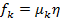It is important to note that, even though this depends on the magnitude of the normal force η, the vector for the force of kinetic friction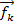is perpendicular to the vector for the normal force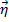,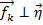When an object is not moving relative to a surface, the friction force it may experience is called static friction. The static friction is written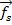. Static friction is different from kinetic friction for two reasons. First, the magnitude of the static friction depends on the force that is attempting to move the object. It can range from zero to a maximum value, beyond which the object starts moving. The second difference is that the maximum value of the static friction can be greater than the kinetic friction. Often, it requires a greater force to start an object moving than it does to keep an object in motion.

The maximum value of the static friction is written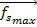. Beyond this value an object begins to move,. If an object is sitting on a surface (for example, a book on a table), and a forceis applied parallel to the surface, then so long as the object does not move, the force of static frictionis,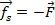The force of static friction is equal in magnitude and opposite in direction to the force that is applied to the object. The force of static friction can have any value between zero and its maximum,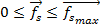The maximum magnitude of the static friction depends on two values. The first is the magnitude of the normal force, η, exerted by the surface. The second value is the "coefficient of static friction". It is represented by the Greek letter μ ("mu"), with the subscript s. The value of μs is often greater than the value of the coefficient of kinetic friction μk.

The maximum force of static friction has magnitude,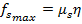Therefore, the static friction has magnitude,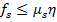It is important to note that, even though this depends on the magnitude of the normal force η, the vector for the force of static frictionis perpendicular to the vector for the normal force,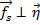Related Links: Physics Quizzes AP Physics Notes Work, Energy, and Power